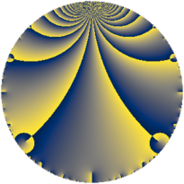# Properties

 Label 6080.2.a.gLevel $6080$ Weight $2$ Character orbit 6080.a Self dual yes Analytic conductor $48.549$ Analytic rank $2$ Dimension $1$ CM no Inner twists $1$

# Related objects

Show commands: Magma / PariGP / SageMath

## Newspace parameters

comment: Compute space of new eigenforms

[N,k,chi] = [6080,2,Mod(1,6080)]

mf = mfinit([N,k,chi],0)

lf = mfeigenbasis(mf)

from sage.modular.dirichlet import DirichletCharacter

H = DirichletGroup(6080, base_ring=CyclotomicField(2))

chi = DirichletCharacter(H, H._module([0, 0, 0, 0]))

N = Newforms(chi, 2, names="a")

//Please install CHIMP (https://github.com/edgarcosta/CHIMP) if you want to run this code

chi := DirichletCharacter("6080.1");

S:= CuspForms(chi, 2);

N := Newforms(S);

 Level: $$N$$ $$=$$ $$6080 = 2^{6} \cdot 5 \cdot 19$$ Weight: $$k$$ $$=$$ $$2$$ Character orbit: $$[\chi]$$ $$=$$ 6080.a (trivial)

## Newform invariants

comment: select newform

sage: f = N # Warning: the index may be different

gp: f = lf \\ Warning: the index may be different

 Self dual: yes Analytic conductor: $$48.5490444289$$ Analytic rank: $$2$$ Dimension: $$1$$ Coefficient field: $$\mathbb{Q}$$ Coefficient ring: $$\mathbb{Z}$$ Coefficient ring index: $$1$$ Twist minimal: no (minimal twist has level 3040) Fricke sign: $$-1$$ Sato-Tate group: $\mathrm{SU}(2)$

## $q$-expansion

comment: q-expansion

sage: f.q_expansion() # note that sage often uses an isomorphic number field

gp: mfcoefs(f, 20)

 $$f(q)$$ $$=$$ $$q - q^{3} - q^{5} - 3 q^{7} - 2 q^{9}+O(q^{10})$$ q - q^3 - q^5 - 3 * q^7 - 2 * q^9 $$q - q^{3} - q^{5} - 3 q^{7} - 2 q^{9} - 5 q^{13} + q^{15} - 3 q^{17} - q^{19} + 3 q^{21} - 7 q^{23} + q^{25} + 5 q^{27} + q^{29} - 2 q^{31} + 3 q^{35} - 2 q^{37} + 5 q^{39} - 10 q^{41} - 6 q^{43} + 2 q^{45} - 8 q^{47} + 2 q^{49} + 3 q^{51} - 9 q^{53} + q^{57} + 5 q^{59} - 4 q^{61} + 6 q^{63} + 5 q^{65} - q^{67} + 7 q^{69} - 12 q^{71} - 13 q^{73} - q^{75} + 6 q^{79} + q^{81} + 18 q^{83} + 3 q^{85} - q^{87} + 2 q^{89} + 15 q^{91} + 2 q^{93} + q^{95} - 14 q^{97}+O(q^{100})$$ q - q^3 - q^5 - 3 * q^7 - 2 * q^9 - 5 * q^13 + q^15 - 3 * q^17 - q^19 + 3 * q^21 - 7 * q^23 + q^25 + 5 * q^27 + q^29 - 2 * q^31 + 3 * q^35 - 2 * q^37 + 5 * q^39 - 10 * q^41 - 6 * q^43 + 2 * q^45 - 8 * q^47 + 2 * q^49 + 3 * q^51 - 9 * q^53 + q^57 + 5 * q^59 - 4 * q^61 + 6 * q^63 + 5 * q^65 - q^67 + 7 * q^69 - 12 * q^71 - 13 * q^73 - q^75 + 6 * q^79 + q^81 + 18 * q^83 + 3 * q^85 - q^87 + 2 * q^89 + 15 * q^91 + 2 * q^93 + q^95 - 14 * q^97

## Embeddings

For each embedding $$\iota_m$$ of the coefficient field, the values $$\iota_m(a_n)$$ are shown below.

For more information on an embedded modular form you can click on its label.

comment: embeddings in the coefficient field

gp: mfembed(f)

Label $$\iota_m(\nu)$$ $$a_{2}$$ $$a_{3}$$ $$a_{4}$$ $$a_{5}$$ $$a_{6}$$ $$a_{7}$$ $$a_{8}$$ $$a_{9}$$ $$a_{10}$$
1.1
 0
0 −1.00000 0 −1.00000 0 −3.00000 0 −2.00000 0
 $$n$$: e.g. 2-40 or 990-1000 Significant digits: Format: Complex embeddings Normalized embeddings Satake parameters Satake angles

## Atkin-Lehner signs

$$p$$ Sign
$$2$$ $$-1$$
$$5$$ $$1$$
$$19$$ $$1$$

## Inner twists

This newform does not admit any (nontrivial) inner twists.

## Twists

By twisting character orbit
Char Parity Ord Mult Type Twist Min Dim
1.a even 1 1 trivial 6080.2.a.g 1
4.b odd 2 1 6080.2.a.q 1
8.b even 2 1 3040.2.a.d yes 1
8.d odd 2 1 3040.2.a.a 1

By twisted newform orbit
Twist Min Dim Char Parity Ord Mult Type
3040.2.a.a 1 8.d odd 2 1
3040.2.a.d yes 1 8.b even 2 1
6080.2.a.g 1 1.a even 1 1 trivial
6080.2.a.q 1 4.b odd 2 1

## Hecke kernels

This newform subspace can be constructed as the intersection of the kernels of the following linear operators acting on $$S_{2}^{\mathrm{new}}(\Gamma_0(6080))$$:

 $$T_{3} + 1$$ T3 + 1 $$T_{7} + 3$$ T7 + 3 $$T_{11}$$ T11

## Hecke characteristic polynomials

$p$ $F_p(T)$
$2$ $$T$$
$3$ $$T + 1$$
$5$ $$T + 1$$
$7$ $$T + 3$$
$11$ $$T$$
$13$ $$T + 5$$
$17$ $$T + 3$$
$19$ $$T + 1$$
$23$ $$T + 7$$
$29$ $$T - 1$$
$31$ $$T + 2$$
$37$ $$T + 2$$
$41$ $$T + 10$$
$43$ $$T + 6$$
$47$ $$T + 8$$
$53$ $$T + 9$$
$59$ $$T - 5$$
$61$ $$T + 4$$
$67$ $$T + 1$$
$71$ $$T + 12$$
$73$ $$T + 13$$
$79$ $$T - 6$$
$83$ $$T - 18$$
$89$ $$T - 2$$
$97$ $$T + 14$$